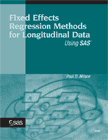As the first step in the decommissioning of sasCommunity.org the site has been converted to read-only mode.

Here are some tips for How to share your SAS knowledge with your professional network.

Fixed Effects Regression Methods for Longitudinal Data Using SAS

OverviewTitle: Fixed Effects Regression Methods for Longitudinal Data Using SAS

Author: Paul D. Allison

ISBN:1590475682

Click on the image to order the book from SAS online bookstore.

Description

Fixed Effects Regression Methods for Longitudinal Data Using SAS is an invaluable resource for all researchers interested in adding fixed effects regression methods to their tool kit of statistical techniques. First introduced by economists, fixed effects methods are gaining widespread use throughout the social sciences. Designed to eliminate major biases from regression models with multiple observations (usually longitudinal) for each subject (usually a person), fixed effects methods essentially offer control for all stable characteristics of the subjects, even characteristics that are difficult or impossible to measure. This straightforward and thorough text shows you how to estimate fixed effects models with several SAS procedures that are appropriate for different kinds of outcome variables. The theoretical background of each model is explained, and the models are then illustrated with detailed examples using real data. The book contains thorough discussions of the following uses of SAS procedures: PROC GLM for estimating fixed effects linear models for quantitative outcomes, PROC LOGISTIC for estimating fixed effects logistic regression models, PROC PHREG for estimating fixed effects Cox regression models for repeated eventdata, PROC GENMOD for estimating fixed effects Poisson regression models for count data, and PROC CALIS for estimating fixed effects structural equation models. To gain the most benefit from this book, readers should be familiar with multiple linear regression, have practical experience using multiple regression on real data, and be comfortable interpreting the output from a regression analysis. An understanding of logistic regression and Poisson regression is a plus. Some experience with SAS is helpful, but not required.